# 数据挖掘实战—航空公司客户价值分析

## 引言

企业在面向客户制定运营策略与营销策略时，希望针对不同的客户推行不同的策略，实现精准化运营。通过客户分类，对客户群体进行细分，区分出低价值客户与高价值客户，对不同的客户群体开展不同的个性化服务，将有限的资源合理地分配给不同价值的客户，从而实现效益（利润）最大化。本文将使用航空公司客户数据，结合RFM模型，采用K-Means聚类算法，对客户进行分群，比较不同类别客户的价值，从而指定相应的营销策略。定义挖掘目标如下：

• 借助航空公司数据对客户进行分类
• 对不同的客户类别进行特征分析，比较不同类别客户的价值
• 针对不同价值的客户制定相应的营销策略，为其提供个性化服务

1. 抽取航空公司2012年4月1日至2014年3月31日的数据
2. 对抽取的数据进行数据探索分析数据预处理，包括数据缺失值与异常值的探索分析、数据清洗特征构建标准化等操作
3. 基于RFM模型，使用K-means算法进行客户分群
4. 针对模型结果得到不同价值的客户，采用不同的营销手段，提供定制化服务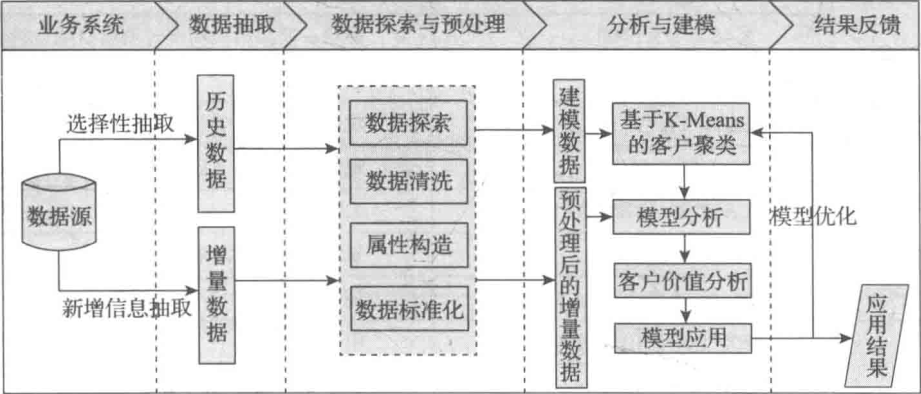## 一、数据探索分析(EDA)

航空公司数据属性说明如下：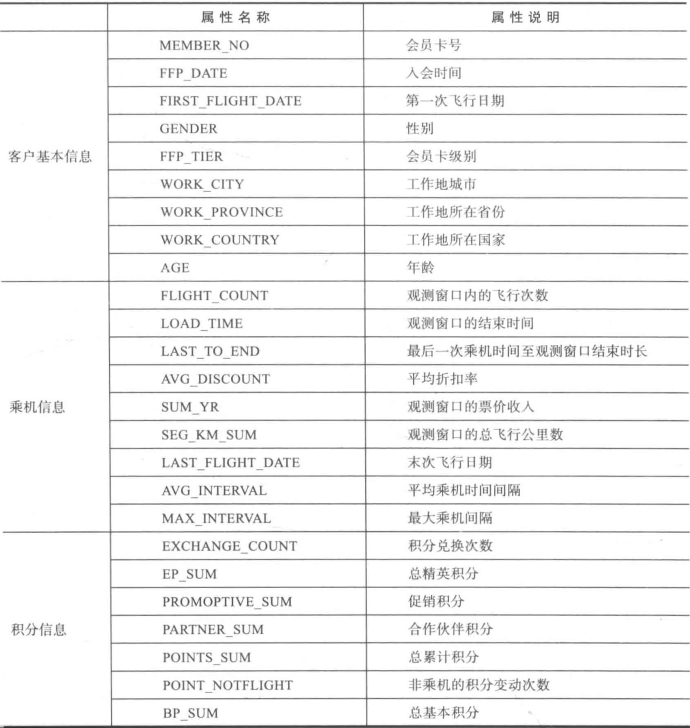%matplotlib inline
import pandas as pd
import numpy as np
import missingno as mg
import matplotlib.pyplot as plt
import seaborn as sns

# 加载数据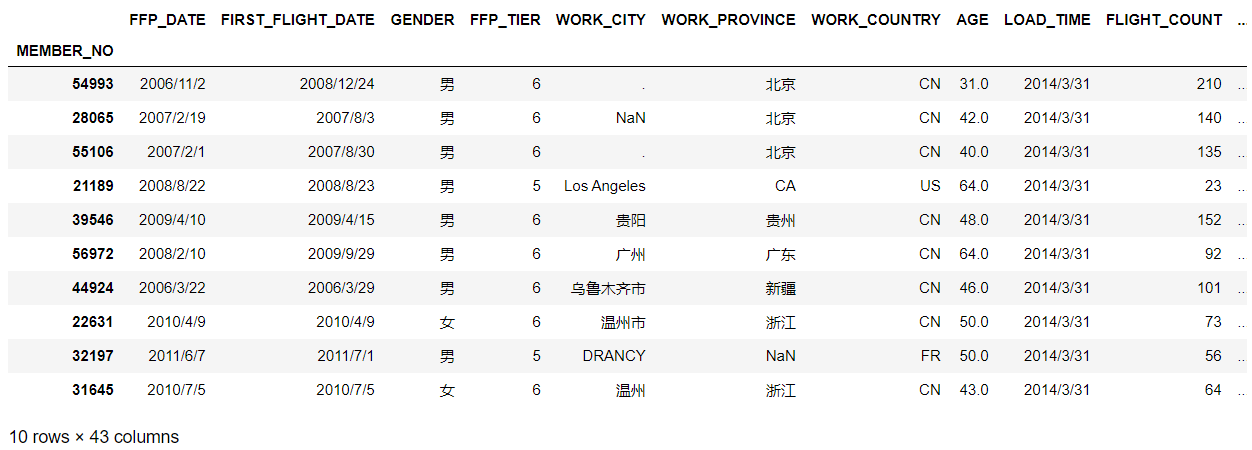### 1.数据质量分析

#### 1.1 缺失值分析

缺失值可视化,图中的白线即为缺失值

# 缺失值可视化
mg.matrix(air_data)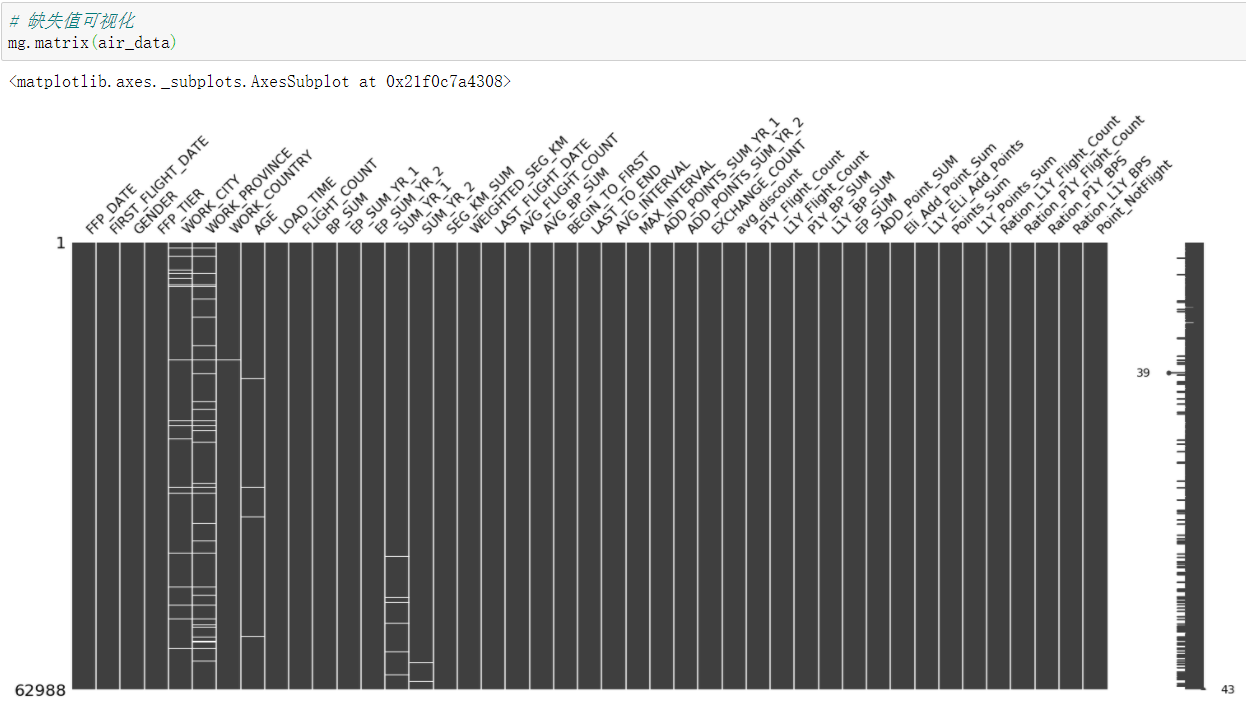利用柱状图进行缺失值可视化，柱状图的高度代表非缺失比例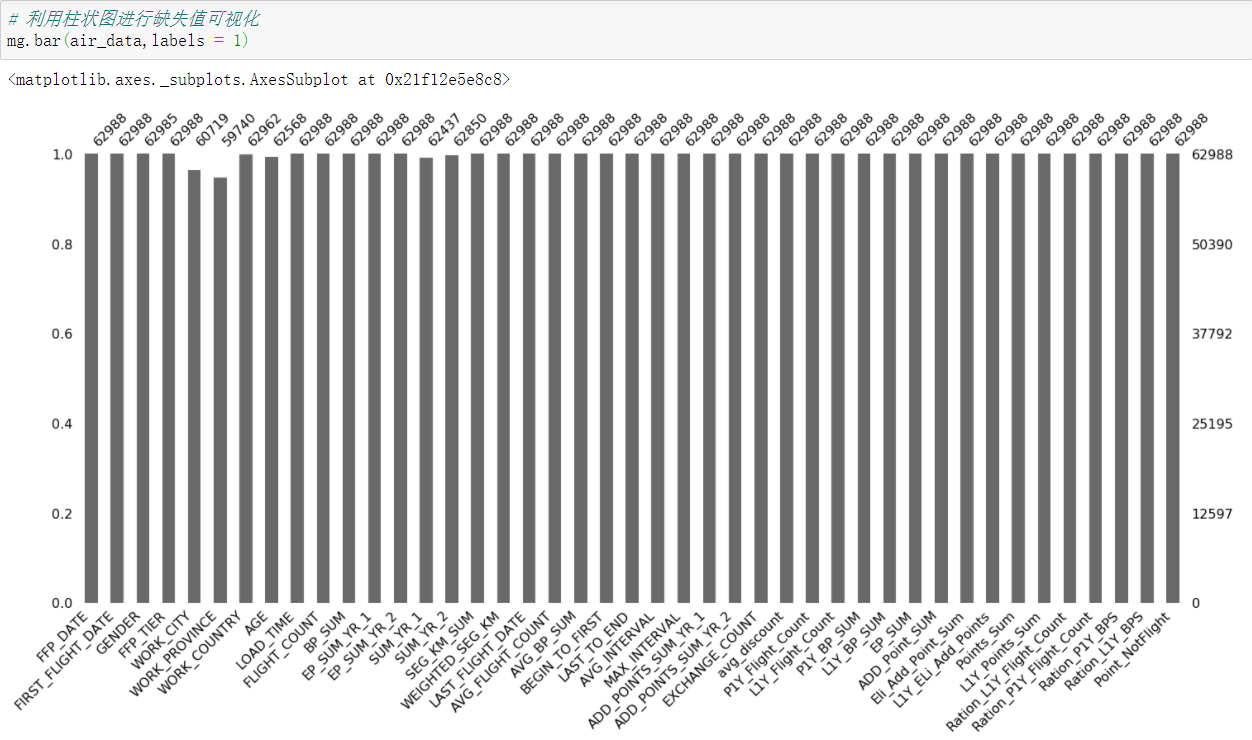定量展示每一列缺失值个数并排序

air_data.isnull().sum().sort_values(ascending=False)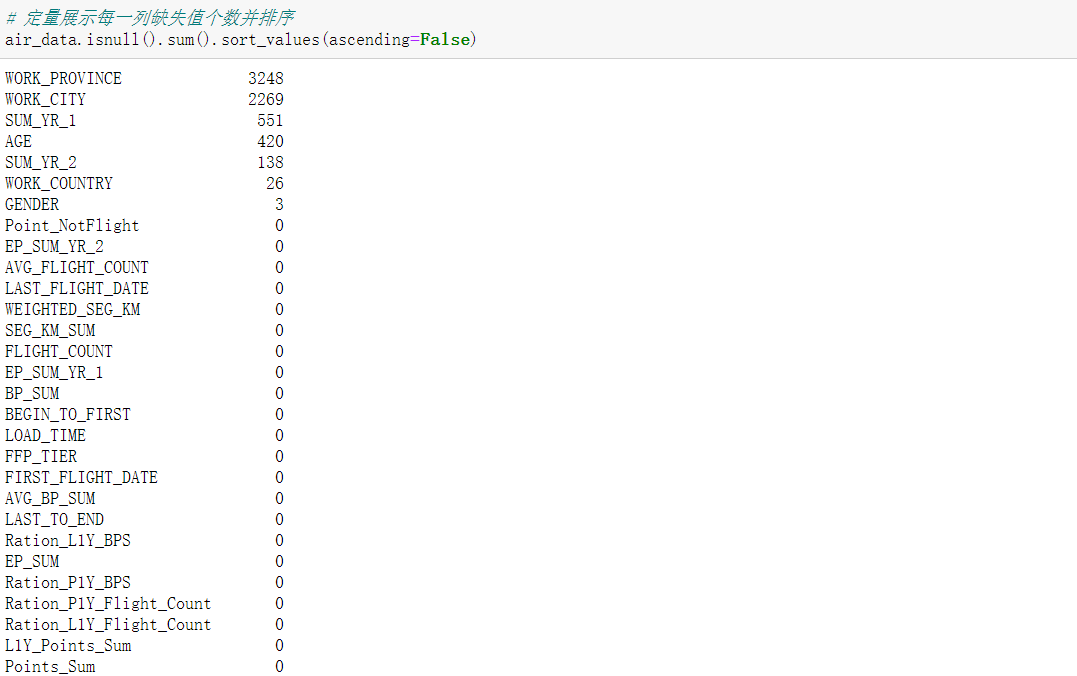展示每一列的缺失值个数与缺失率以及缺失值的属性

def missing_percentage(df):
dtypes = df.dtypes[df.isnull().sum() != 0]
total = df.isnull().sum().sort_values(ascending=False)[df.isnull().sum() != 0]
percentage = total*100 / len(df)
return pd.concat([total,percentage,dtypes],axis=1,keys=['Total','Percentage','Dtypes'])

missing_percentage(air_data)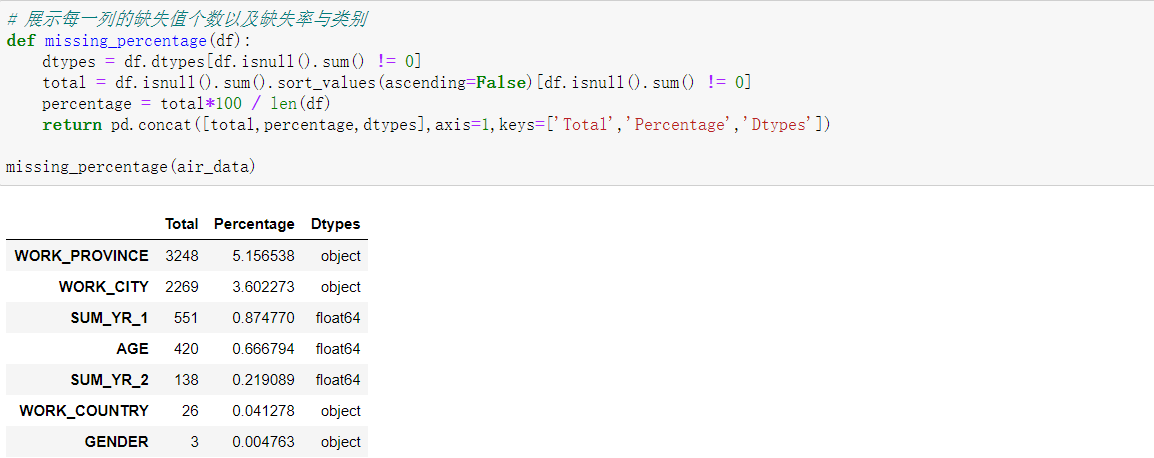#### 1.2 异常值分析

用箱型图来识别异常值，箱型图识别异常值是以四分位数与四分位距为基础，同时结合业务逻辑，因为异常值并不代表是错误值，需要结合业务来考虑异常值在不在合理的范围内。

# 数据类别统计分析
air_data.dtypes.value_counts()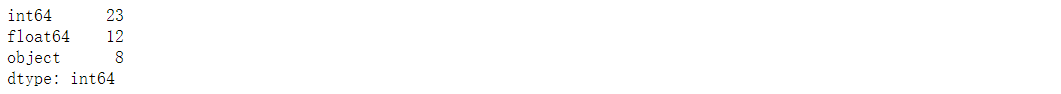# 连续型变量
num_columns = air_data.loc[:,air_data.dtypes != object].columns
for var in num_columns:
fig,ax = plt.subplots(figsize=(5,5))
sns.boxplot(air_data[var],orient='v')
ax.set_xlabel(var)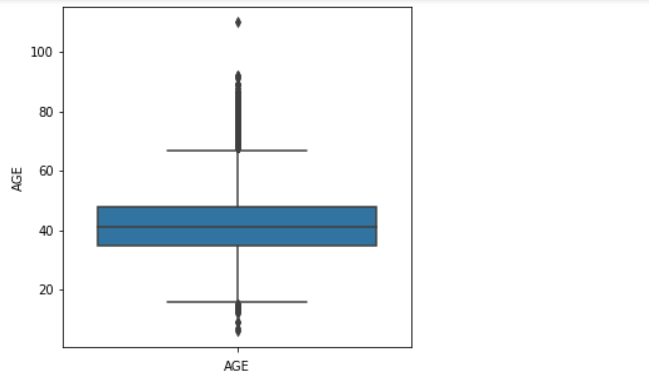对每个图进行分析，分析结果如下，这个是自身对业务的认知水平基础上的分析

FFP_TIER:会员卡级别中数据要么6要么5没有异常值
AGE:年龄中可以看到有些大于100岁，要对这些数据进行处理
FLIGHT_COUNT:观测窗口内的飞行次数没有小于0的值，其他不在箱子中的异常值是有价值
BP_SUM:总基本积分没有小于0的值，其他不在箱子里面的值是有价值
EP_SUM =EP_SUM_YR_1 +EP_SUM_YR_2 :总精英积分有1个值远高于其他值，这个我认为是有价值的异常值
SUM_YR = SUM_YR_1 + SUM_YR_2:观测窗口的票价收入，没有低于0的，有特别高的，我认为是有价值的异常值
SEG_KM_SUM :观测窗口的飞行里程数，没有小于0的值，有特别高,我认为是有价值的异常值
WEIGHTED_SEG_KM : 含义未知，跟里程数有关
AVG_FLIGHT_COUNT : 平均里程数 没有小于0的值，有特别高,我认为是有价值的异常值
AVG_BP_SUM:平均基本积分，没有小于0的，有特别高的，我认为是有价值的异常值
BEGIN_TO_FIRST:我猜测是第一次乘机时间至观测窗口结束时长，没有小于0的，有特别高的，我认为是有价值的异常值
LAST_TO_END:最后一次乘机时间至观测窗口结束时长，我猜测是时间间隔类数据，没有小于0的，有特别高的，我认为是有价值的异常值
AVG_INTERVAL : 平均乘机时间间隔，即(LAST_TO_END - BEGIN_TO_FIRST) / FLIGHT_COUNT，没有负值
MAX_INTERVAL : 最大乘机时间间隔，没有负值
EXCHANGE_COUNT : 积分兑换次数，没有负值
Ponits_Sum : 总累积积分，看着没有小于0的，有特别高的，我认为是有价值的异常值
Ponit_NotFlight:非乘机的积分变动次数，看着没有小于0的

#### 1.3 重复数据分析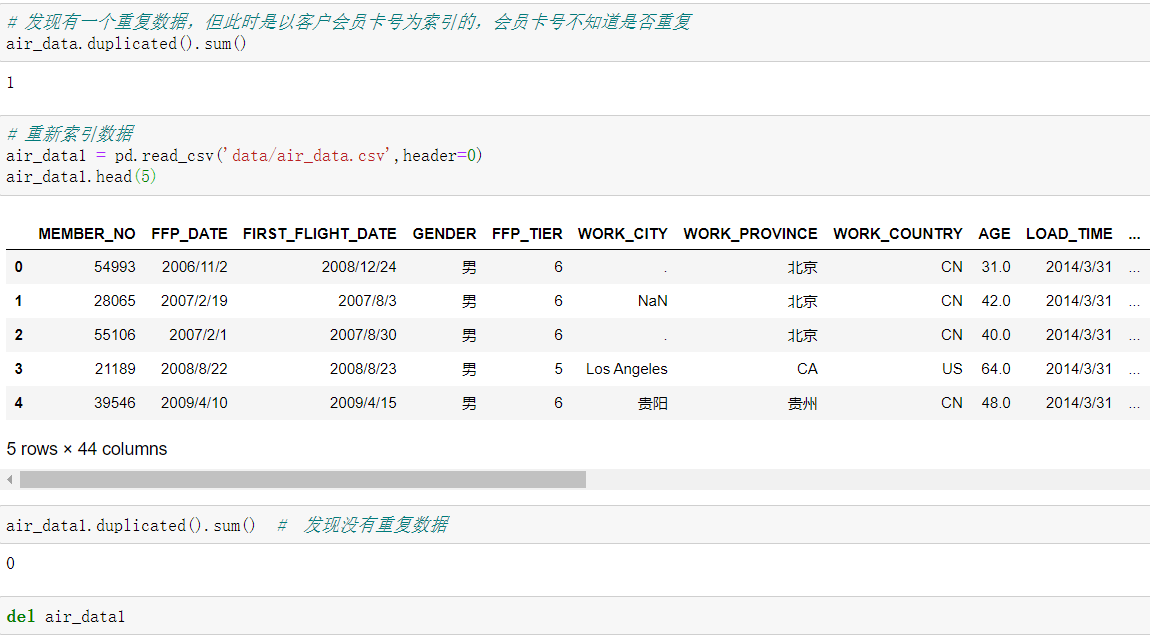### 2.数据特征分析

#### 2.1 描述性统计分析

explore = air_data.describe().T
# count代表非空值个数
explore['null'] = len(air_data) - explore['count']
# 构建缺失值个数，最大值，最小值的表格
explore = explore[['null','min','max']]
explore.columns = ['空值个数','最小值','最大值']
# 按空值个数进行排序
explore.sort_values(by='空值个数',ascending= False,inplace=True)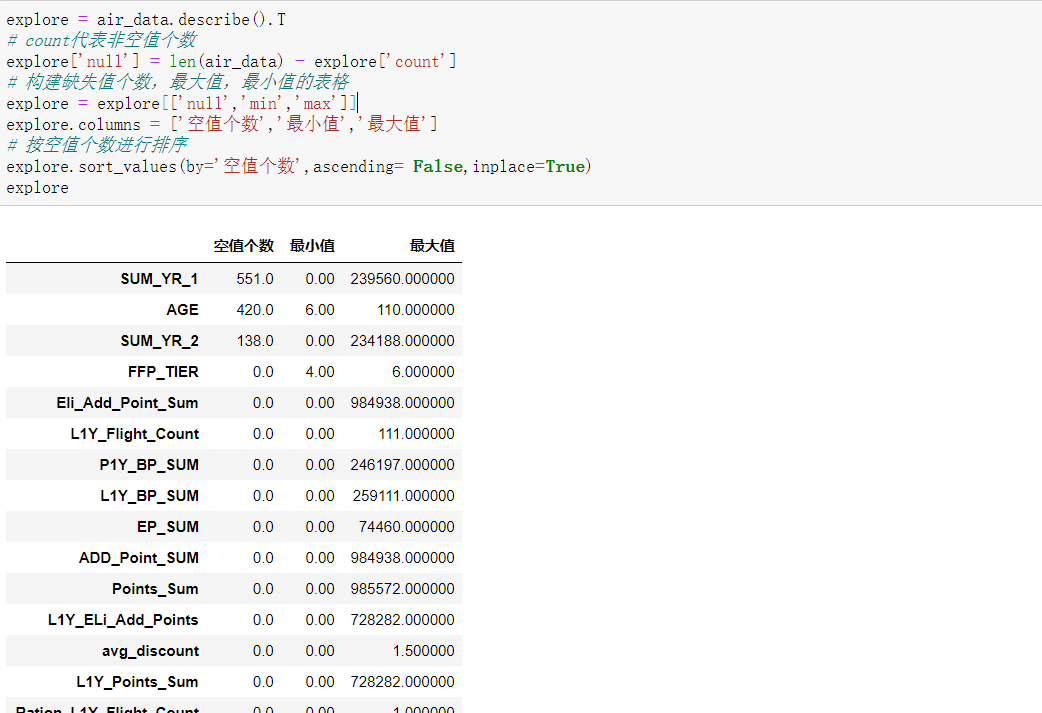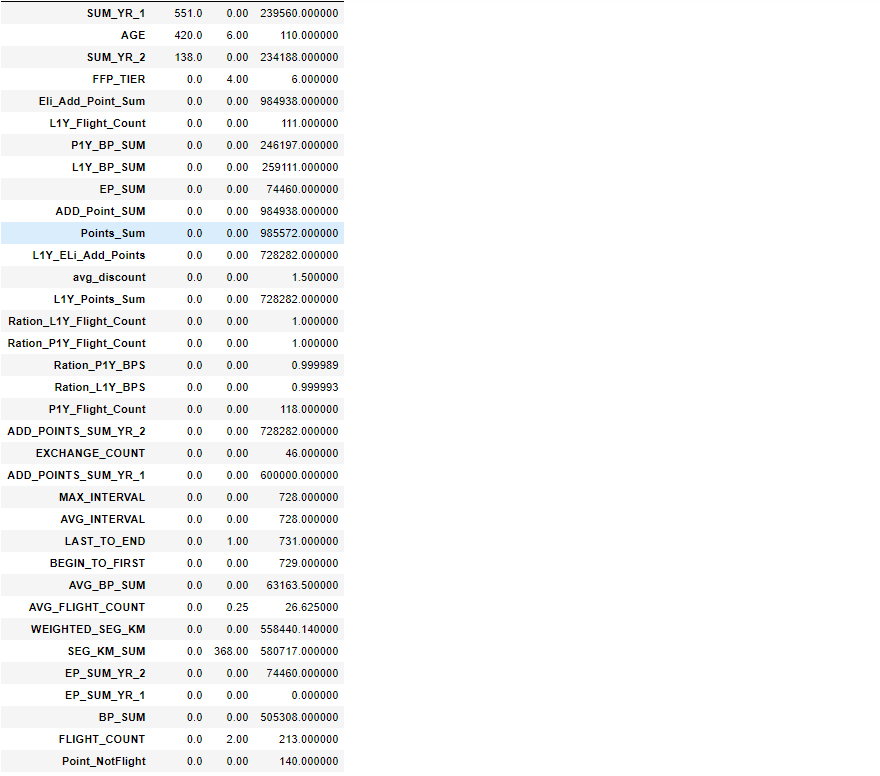从表中可以看出，有3个连续型数据列有缺失值，年龄最大值为110岁，年龄中存在异常值。在三个含缺失值列中有两个是票价(SUM_YR = SUM_YR_1 + SUM_YR_2)，票价为空值的数据可能是客户不存在乘机记录造成的。票价列还存在票价为0，折扣率为0，但总飞行里程数大于0的记录。这种数据业务上可以这么理解：客户乘坐了0折机票或者机票是通过非购买的其他途径获得。

#### 2.2 分布分析

从三个角度寻找客户信息的分布规律

##### 2.2.1 客户基本信息分布分析

针对客户基本信息中的入会时间、性别、会员卡级别和年龄字段进行分析

1. 入会时间—各年份入会人数变化图
from datetime import datetime

# 将时间字符串转换为日期
ffp = air_data['FFP_DATE'].apply(lambda x : datetime.strptime(x,'%Y/%m/%d'))
# 提取入会年份
ffp_year = ffp.map(lambda x : x.year)
# 统计人数
ffp_year_count = ffp_year.value_counts()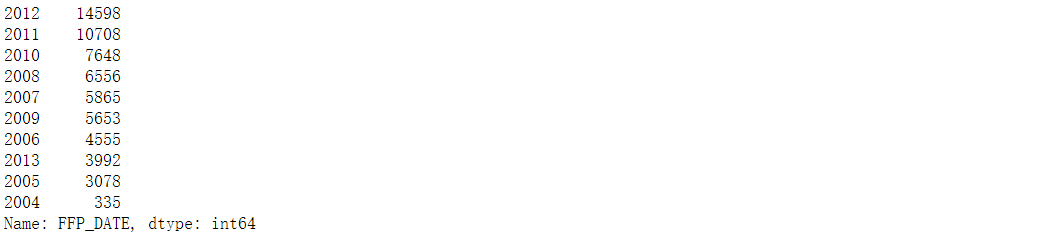# 绘制各年份入会人数趋势图
plt.rcParams['font.sans-serif'] = [u'simHei']   # 显示中文
plt.rcParams['axes.unicode_minus'] = False      # 解决负号问题

plt.figure(figsize=(10,10))
plt.hist(ffp_year,bins='auto',color='r')
plt.xlabel('年份')
plt.ylabel('入会人数')
plt.title('各年份入会人数变化趋势图')
plt.show()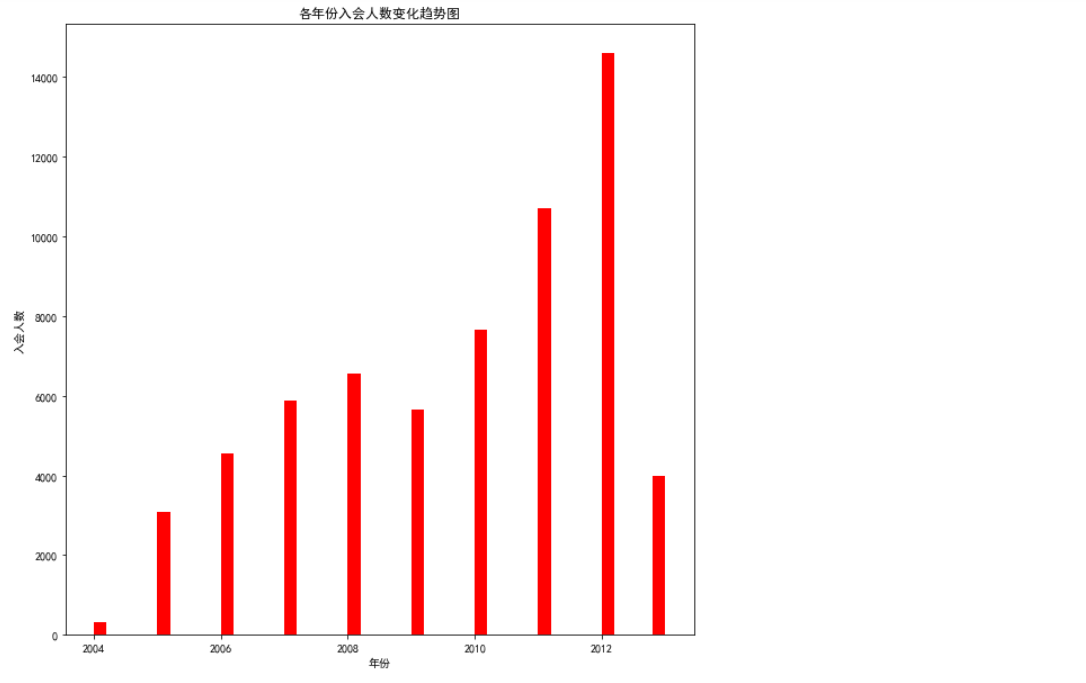1. 性别—入会性别比例图
gender =  air_data['GENDER']
# 统计男女比例
gender_count = gender.value_counts()
gender_count = gender_count/gender_count.sum()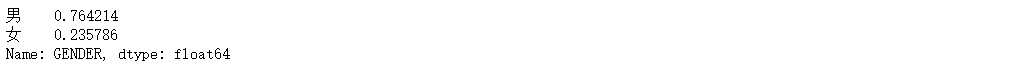plt.figure(figsize=(8,8))
color = ['yellowgreen', 'gold']
plt.pie(gender_count,labels=['男','女'],colors=color,autopct='%1.1f%%')
plt.title('入会性别比例图',fontsize=20)
plt.show()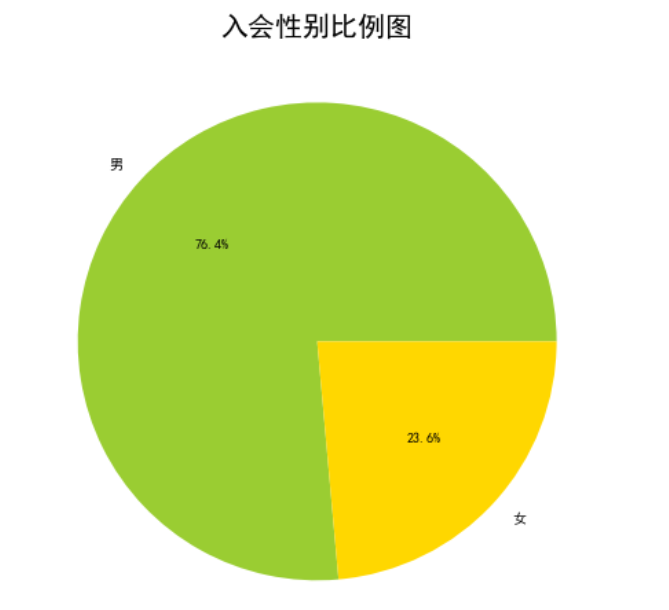1. 会员卡级别—会员卡级别统计图
ffp_tier = air_data['FFP_TIER']
# 会员卡级别统计
ffp_tier_count = ffp_tier.value_counts()
ffp_tier_count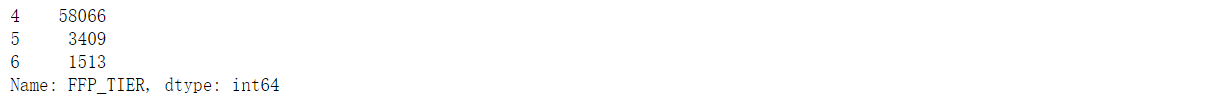plt.figure(figsize=(8,8))
plt.hist(ffp_tier,bins='auto',color='b',alpha=0.8)
plt.xlabel('会员卡级别',fontsize=15)
plt.ylabel('人数',fontsize=15)
plt.title('会员卡级别统计图',fontsize=20)
plt.show()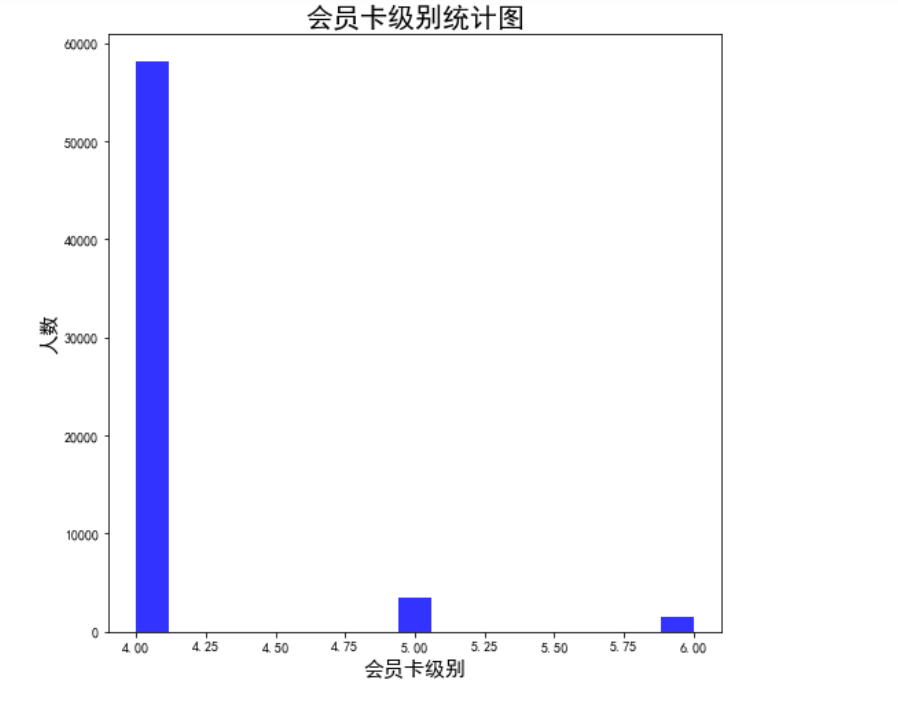1. 年龄
age = air_data['AGE'].dropna()
# 绘制箱型图
plt.figure(figsize=(10,10))
sns.boxplot(age,orient='v')
plt.xlabel('会员年龄',fontsize=15)
plt.title('会员年龄分布箱型图',fontsize=20)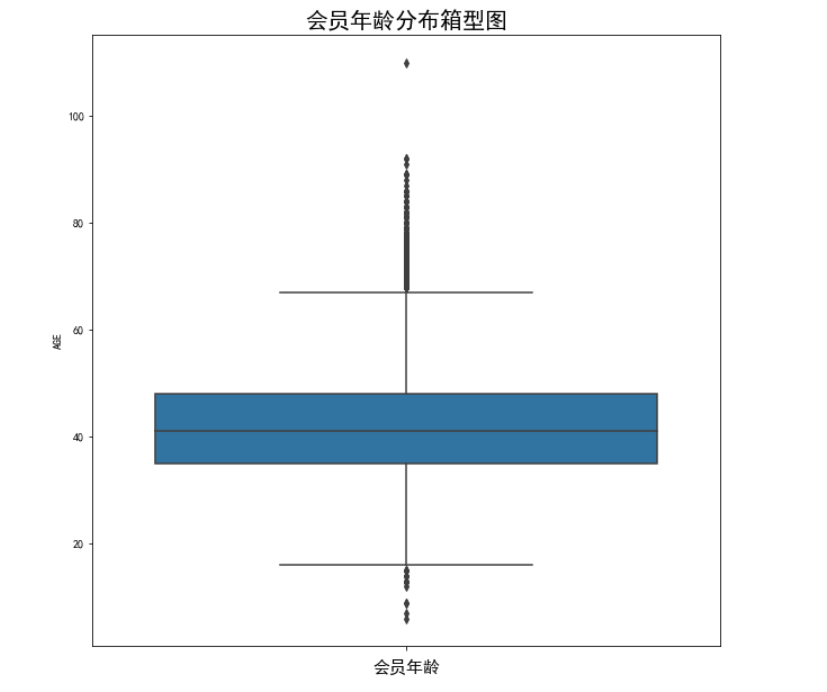##### 2.2.2 客户乘机信息分析

针对客户乘机信息中的观测窗口内的飞行次数，观测窗口内的总飞行公里数，观测窗口内的票价收入，平均乘机时间间隔来分析

1. 观测窗口内的飞行次数与观测窗口内的总飞行公里数
flight_count = air_data['FLIGHT_COUNT']
# 统计飞行次数
flight_count.value_counts()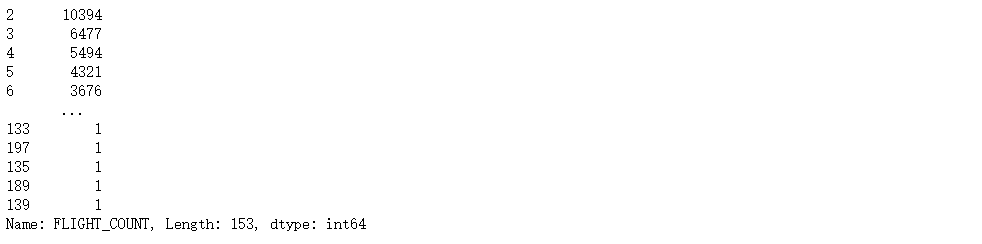seg_km_sum = air_data['SEG_KM_SUM']
# 统计飞行里程数
seg_km_sum.value_counts()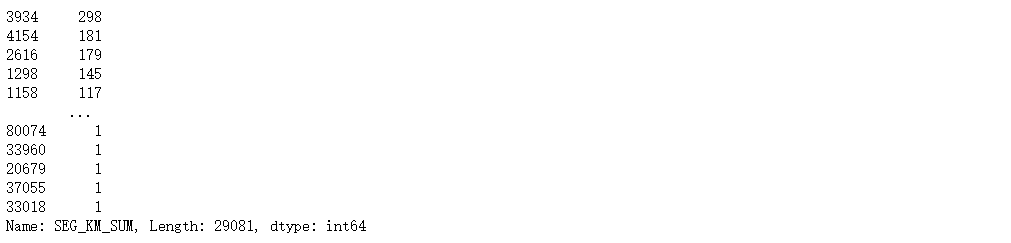对观测窗口内的飞行次数与观测窗口内的总飞行公里数进行绘图

fig,ax = plt.subplots(1,2,figsize=(16,8))
sns.boxplot(flight_count,orient='v',ax=ax)
ax.set_xlabel('飞行次数',fontsize=15)
ax.set_title('会员飞行次数分布箱型图',fontsize=20)

sns.boxplot(seg_km_sum,orient='v',ax=ax)
ax.set_xlabel('飞行里程数',fontsize=15)
ax.set_title('会员飞行里程数分布箱型图',fontsize=20)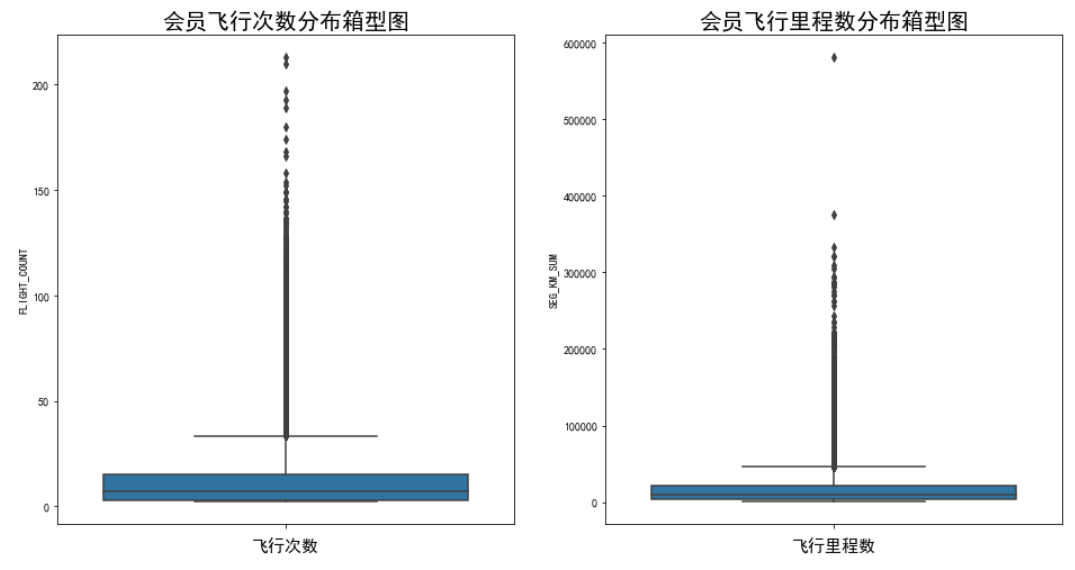1. 观测窗口内的票价收入
sum_yr = air_data['SUM_YR_1'] + air_data['SUM_YR_2']
sum_yr.value_counts()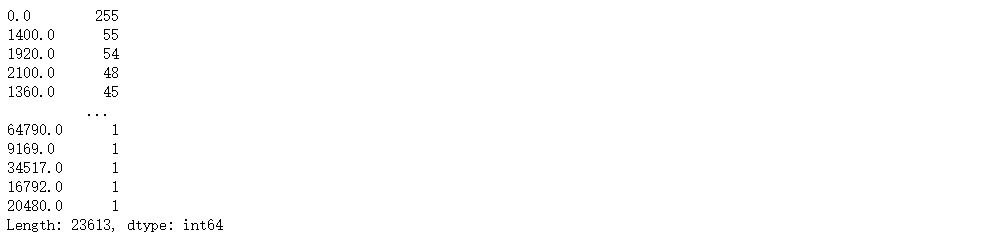plt.figure(figsize=(10,10))
sns.boxplot(sum_yr,orient='v')
plt.xlabel('票价收入',fontsize=15)
plt.title('会员票价收入分布箱型图',fontsize=20)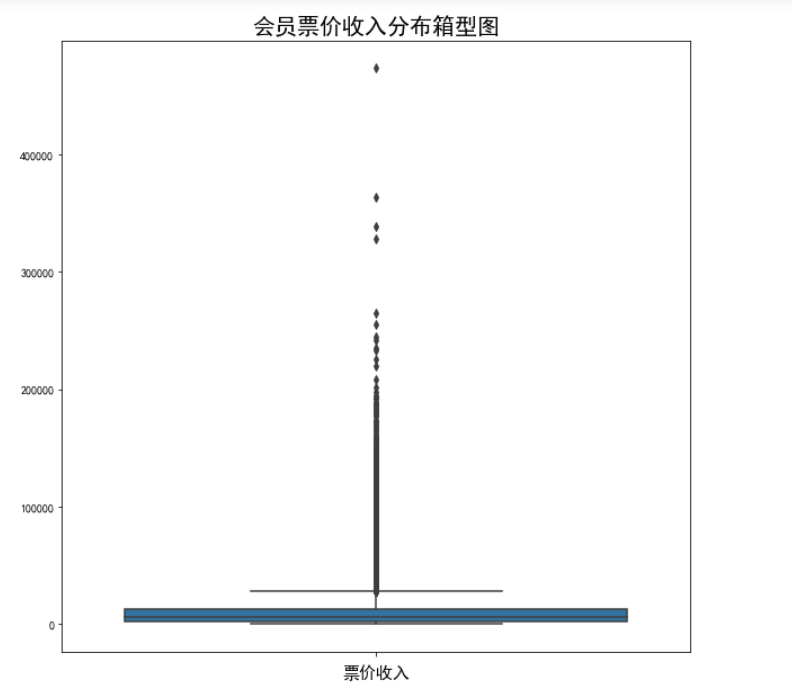1. 平均乘机时间间隔统计
avg_interval = air_data['AVG_INTERVAL']
avg_interval.value_counts()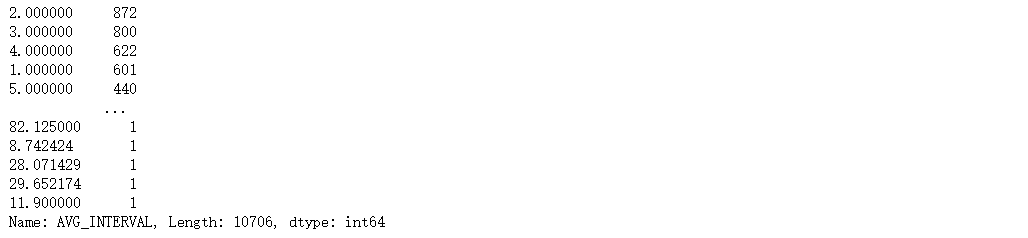plt.figure(figsize=(10,10))
sns.boxplot(avg_interval,orient='v')
plt.xlabel('平均乘机时间间隔',fontsize=15)
plt.title('会员平均乘机时间间隔分布箱型图',fontsize=20)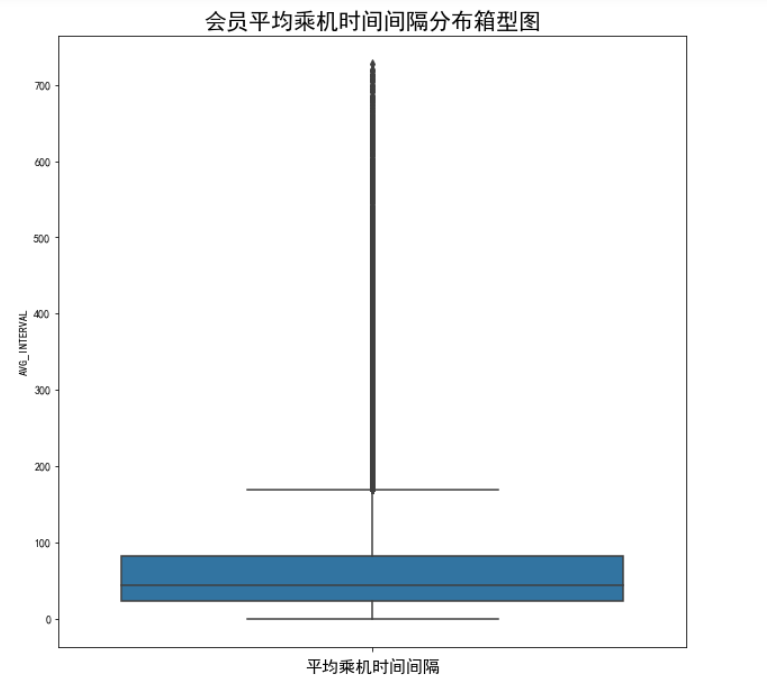1. 最后一次乘机时间至观测窗口时长
last_to_end = air_data['LAST_TO_END']
plt.figure(figsize=(8,8))
sns.boxplot(last_to_end,orient='v')
plt.xlabel('最后一次乘机时间至观测窗口时长',fontsize=15)
plt.title('客户最后一次乘机时间至观测窗口时长箱型图分布',fontsize=20)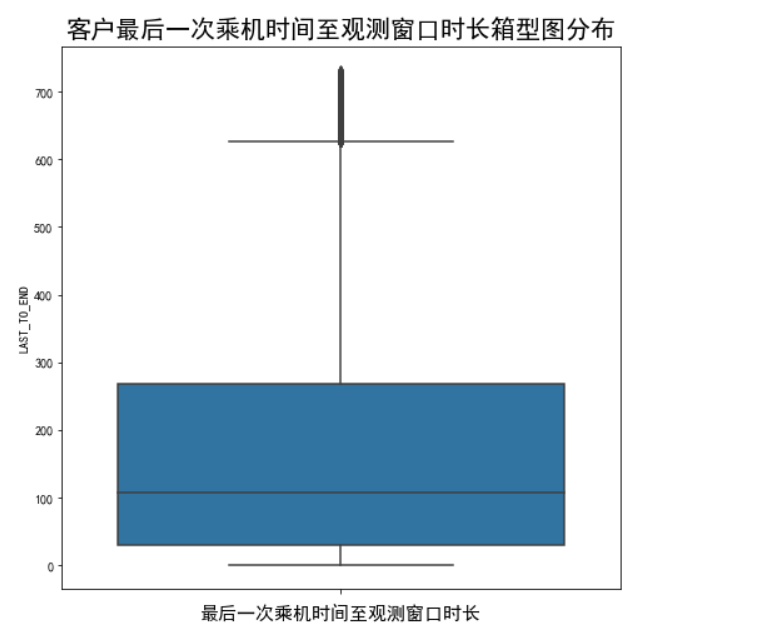##### 2.2.3 客户积分信息分布分析

针对客户积分信息中的积分兑换次数、总累计积分进行分析

1. 积分兑换次数
exchange_count = air_data['EXCHANGE_COUNT']
# 统计积分兑换次数
exchange_count.value_counts()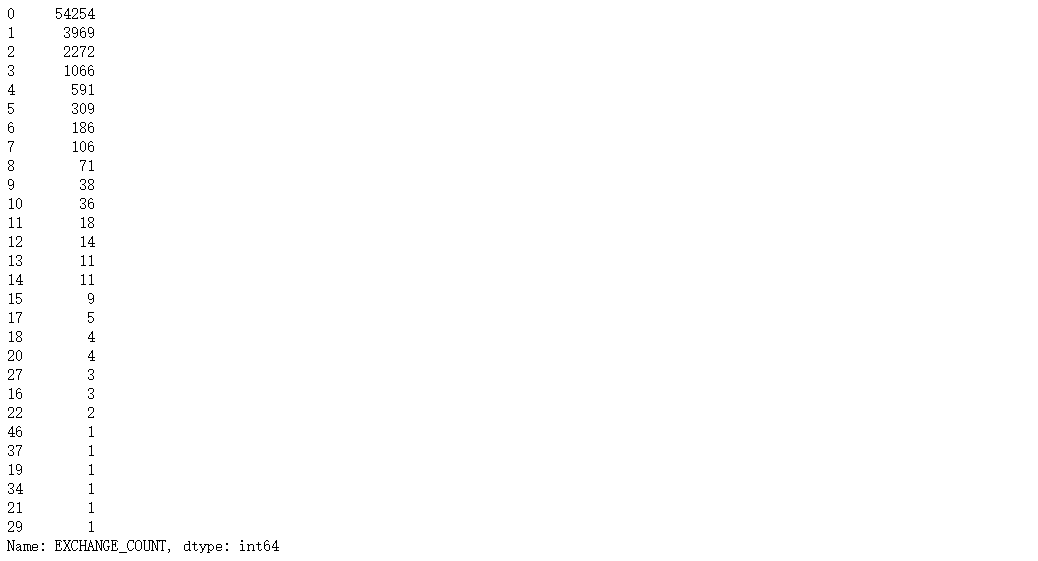plt.figure(figsize=(8,8))
plt.hist(exchange_count,bins='auto',color='b',alpha=0.8)
plt.xlabel('积分兑换次数',fontsize=15)
plt.ylabel('会员人数',fontsize=15)
plt.title('会员卡积分兑换次数分布直方图',fontsize=20)
plt.show()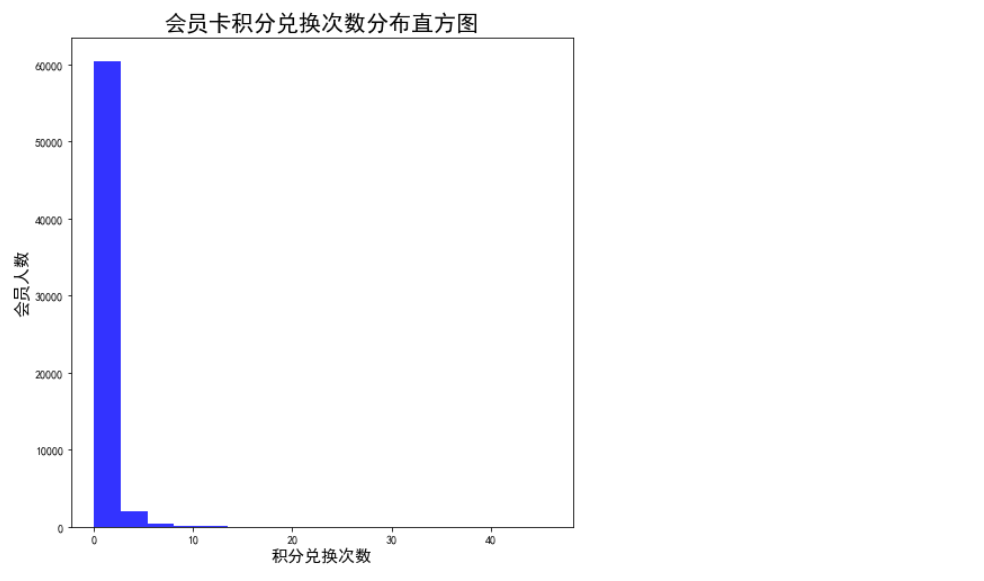2. 总累计积分

point_sum= air_data['Points_Sum']
# 统计总累计积分
point_sum.value_counts()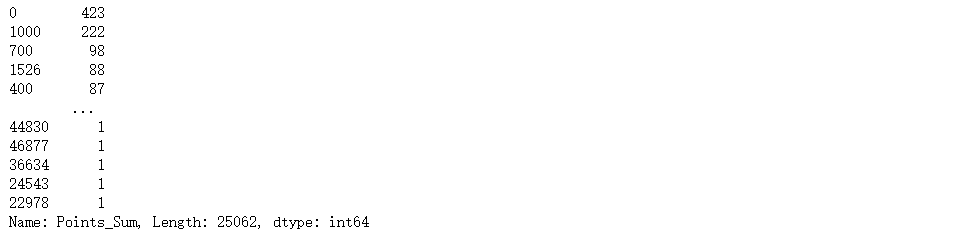plt.figure(figsize=(10,10))
sns.boxplot(point_sum,orient='v')
plt.xlabel('总累计积分',fontsize=15)
plt.title('会员总累计积分分布箱型图',fontsize=20)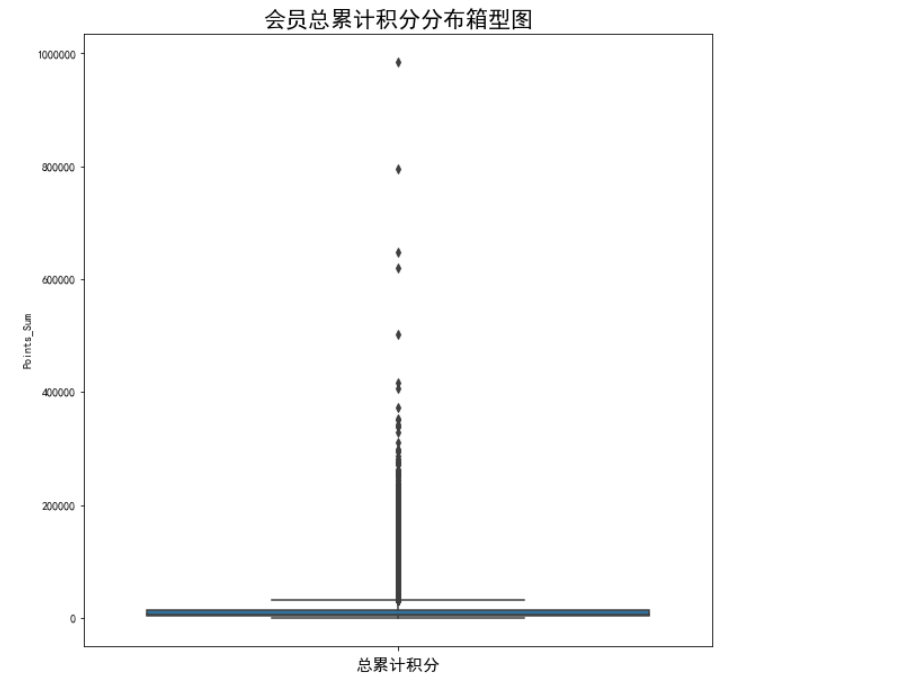#### 2.3 相关性分析

corr = air_data.corr()
# 画热力图
fig,ax = plt.subplots(figsize=(16,16))
sns.heatmap(corr,
annot=True,
square=True,
center=0,
ax=ax)
plt.title("Heatmap of all the Features", fontsize = 30)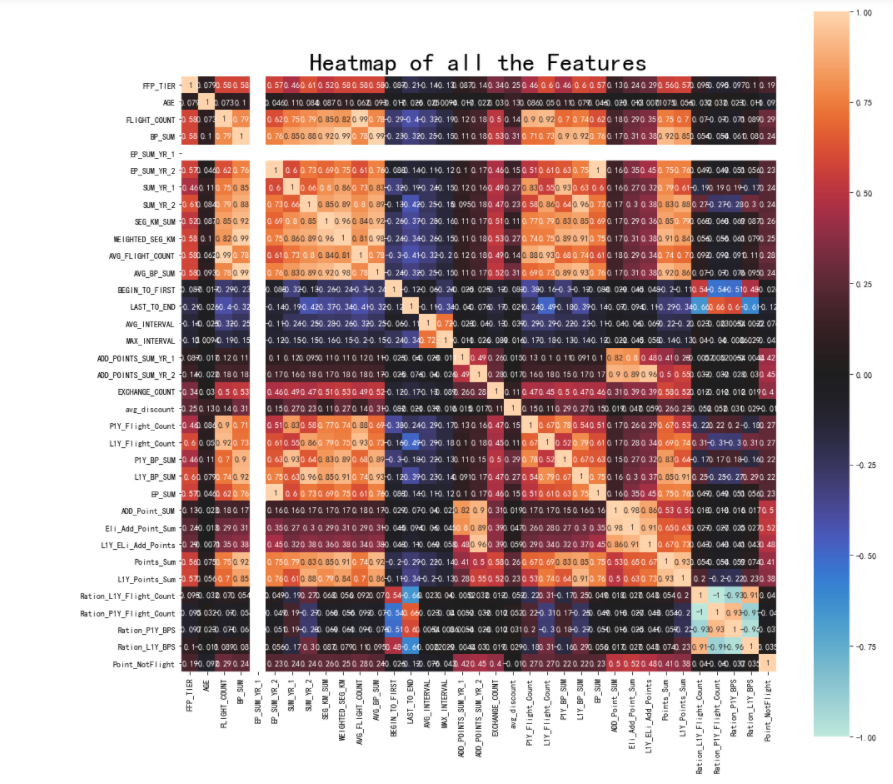分析入会时间、会员卡级别、会员年龄、飞行次数、飞行里程数、观测窗口内的票价收入，平均乘机时间间隔，最近一次乘机至结束时长、积分兑换次数、总累计积分属性的相关性

data_corr = air_data[['FFP_TIER','FLIGHT_COUNT','LAST_TO_END','SEG_KM_SUM','AVG_INTERVAL','EXCHANGE_COUNT','Points_Sum']]
age1 = air_data['AGE'].fillna(0)
data_corr['AGE'] = age1.astype('int64')
data_corr['ffp_year'] = ffp_year
data_corr['sum_yr'] = sum_yr.fillna(0)
data_corr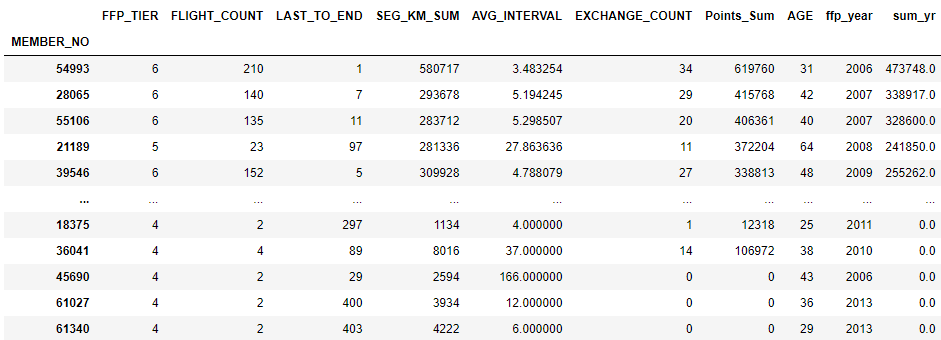# 计算相关性矩阵
dt_corr = data_corr.corr(method='pearson')
dt_corr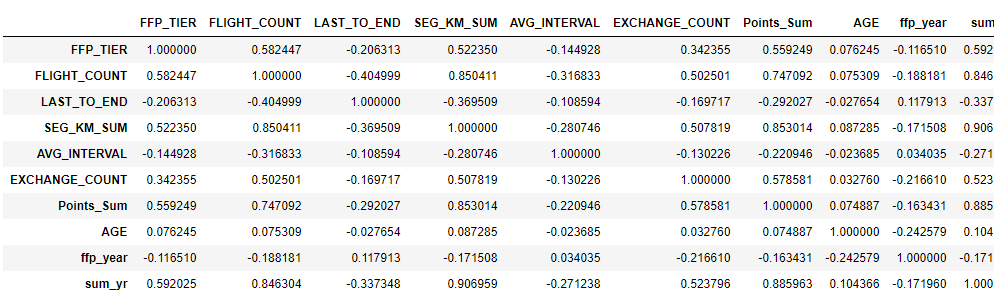fig,ax = plt.subplots(figsize=(16,16))
# 绘制热力图
sns.heatmap(dt_corr,
annot=True,
square= True,
center=0,
ax=ax)
plt.title("Heatmap of some Features", fontsize = 30)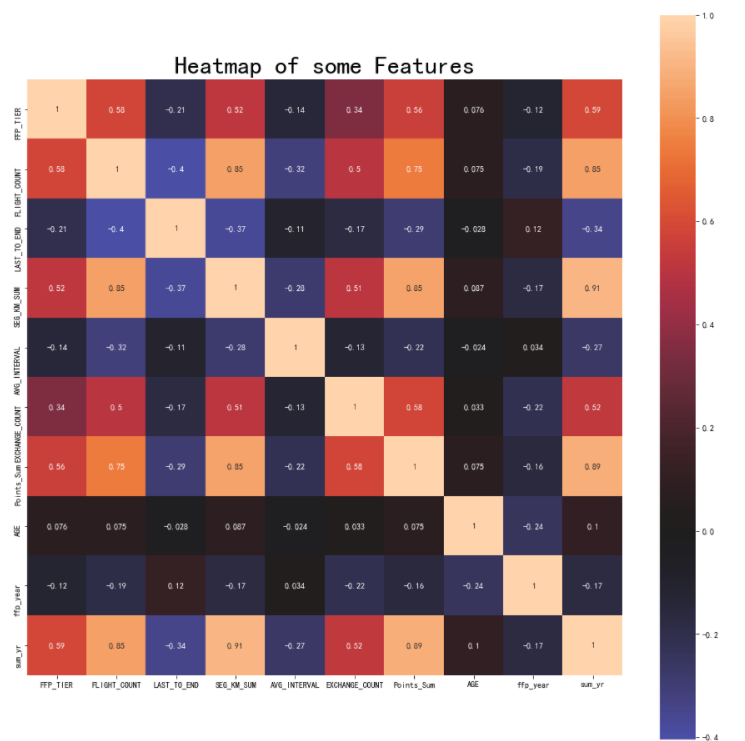## 二、数据预处理

针对航空客户数据从数据清洗、属性归纳与数据变换入手进行数据预处理

### 1.数据清洗

#### 1.1 异常值处理

根据数据预处理我们知道了，原始数据中存在票价为空值的记录，同时存在票价最小值为0，折扣率最小值为0，总飞行公里数大于0的记录，同时年龄中存在一个大于100的记录。由于这类数据在原始数据中占比不大，这里采用丢弃处理。

# 删除年龄中的异常值
print(data.shape)
# 去除票价为空的记录
data_notnull = data.loc[data['SUM_YR_1'].notnull() & data['SUM_YR_2'].notnull(),:]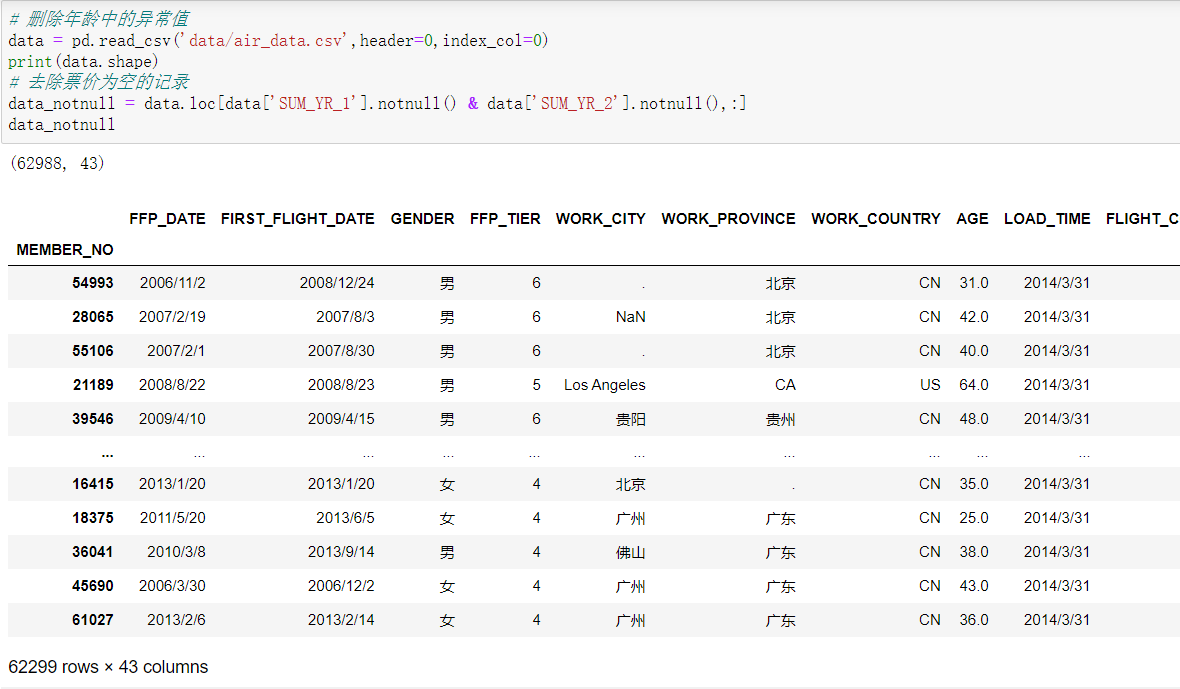# 只保留票价非零的，或者平均折扣率不为0且总飞行公里数大于0的记录,去除年龄大于100的记录
index1 = data_notnull['SUM_YR_1'] !=0
index2 = data_notnull['SUM_YR_2'] !=0
index3 = (data_notnull['avg_discount'] !=0) & (data_notnull['SEG_KM_SUM'] >0)
index4 = data_notnull['AGE'] >100

airline = data_notnull[(index1|index2 ) & index3 & ~index4]   # 按位取反
airline.to_csv('data_cleaned.csv')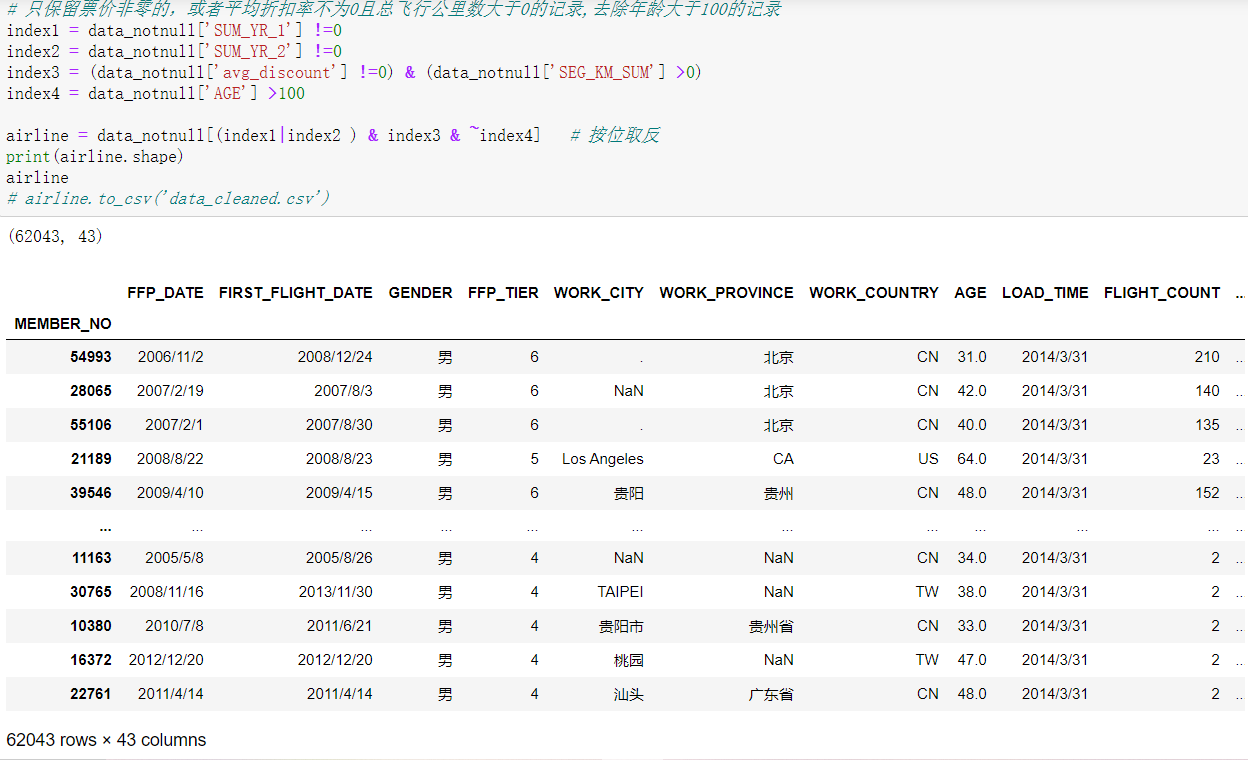#### 1.2 缺失值处理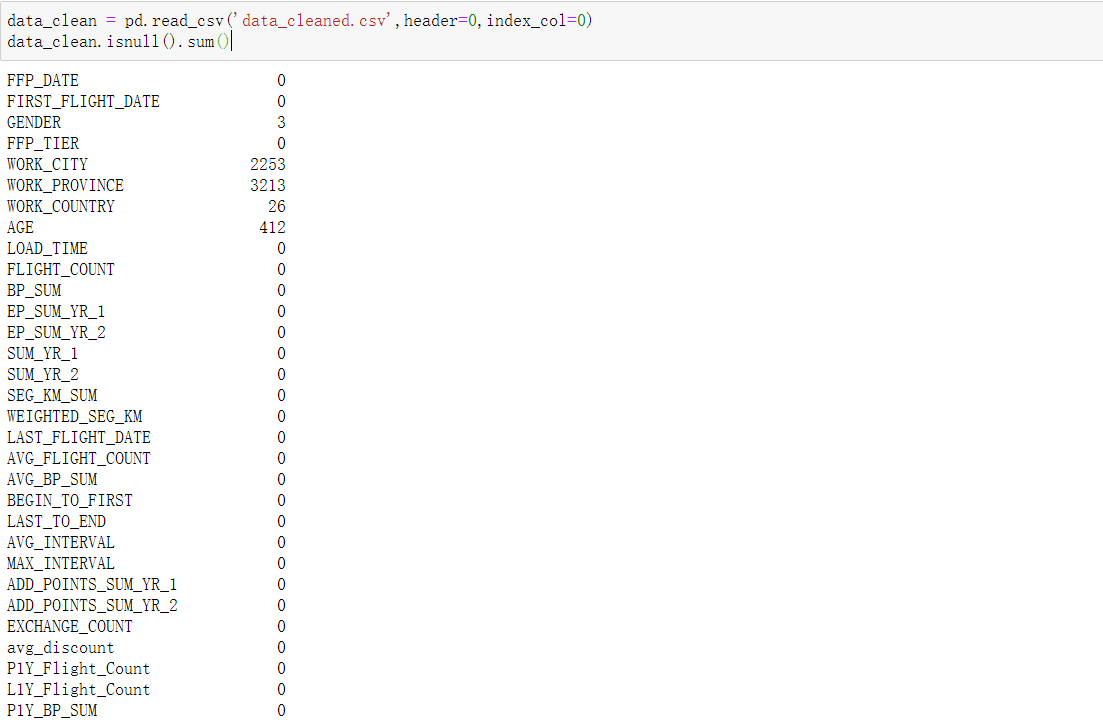1个连续型数据：AGE有缺失值

data_clean['AGE'].fillna(data_clean['AGE'].mean(),inplace = True)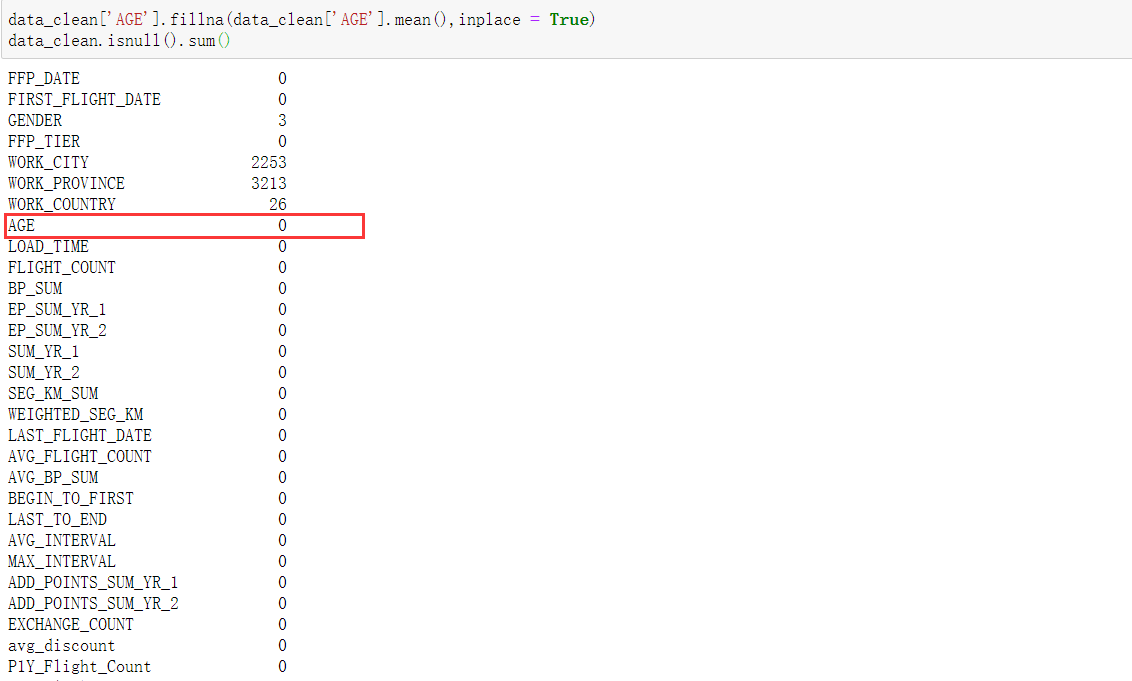### 2.属性归约

RFM模型中，消费金额(M)表示一段时间内客户购买该企业产品金额的总和。由于航空公司票价受运输距离、舱位等级等影响因素，即并不是金额越高的客户并不一定比金额低的客户价值高（长途经济舱与短途商务舱的对比）。基于航空公司业务，这个特征用一定时间内累计的飞行里程M 与客户在一定时间内乘坐舱位对应的平均折扣率C来代替。同时考虑到会员的入会时间在一定程度上能够影响客户的价值，所以在模型中增加客户关系长度L，作为区分客户的一种特征。本模型将以下5个特征作为识别客户价值的特征，即为LRFMC模型。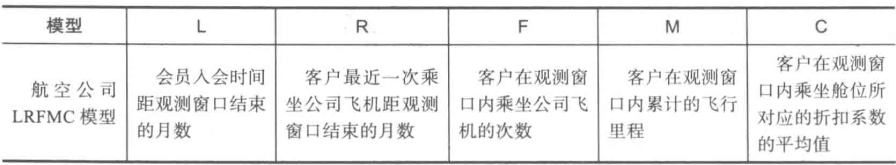data_select = data_clean[['FFP_DATE','LOAD_TIME','LAST_TO_END', 'FLIGHT_COUNT','SEG_KM_SUM','avg_discount']]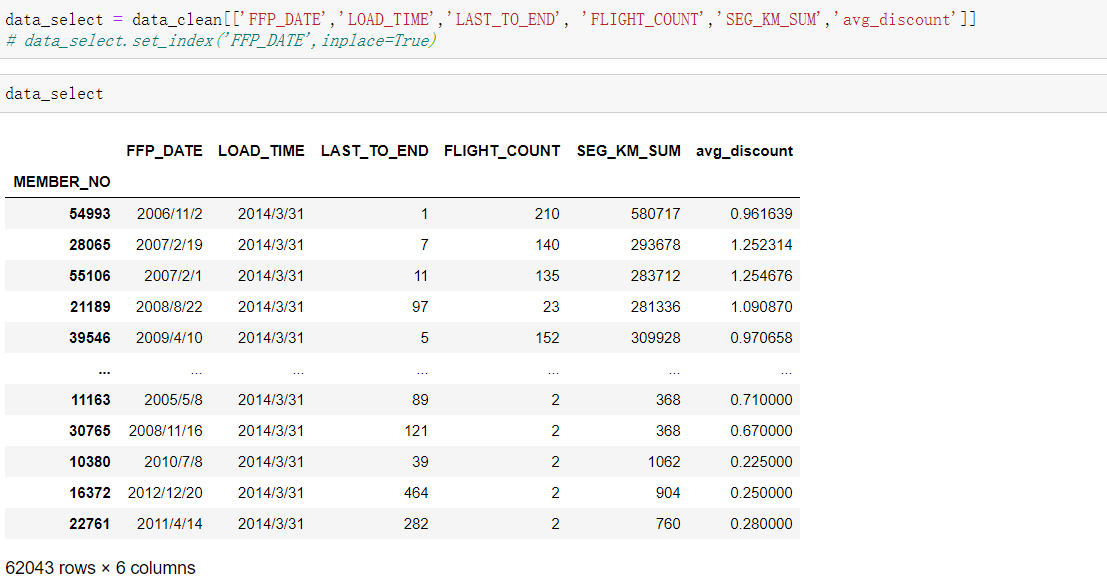### 3.数值变换

构造入会时长指标，并对数据进行标准化

# 构造L 单位为天数
L = L.astype('str').str.split().str   # 去掉单位

# 构造LRFMC指标
data_change = pd.concat([L,data_select.iloc[:,2:]],axis=1)
data_change.columns = ['L','R','F','M','C']

# 标准化—聚类模型基于距离
from sklearn.preprocessing import StandardScaler
data_scale = StandardScaler().fit_transform(data_change)
# 保存数据
np.savez('data_scale.npz',data_scale)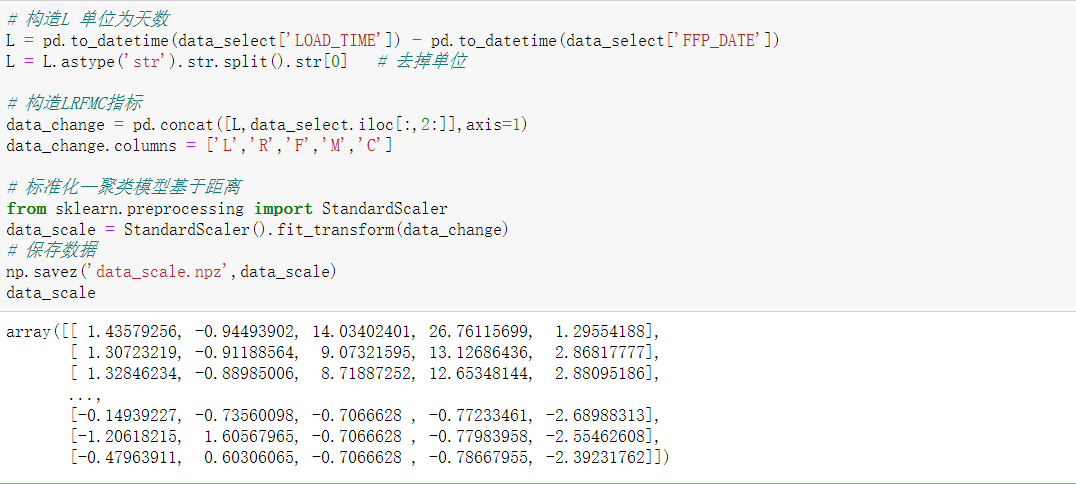## 三、模型构建

### 1.客户聚类分群

采用Kmeans算法对客户进行聚类，基于业务逻辑，聚类为5群

from sklearn.cluster import KMeans
kmeans = KMeans(n_clusters=5,n_jobs=-1,random_state=1234)
# 模型训练
kmeans_fit = kmeans.fit(data_scale)
# 聚类中心
kmeans_cluster = kmeans_fit.cluster_centers_
print('聚类中心为\n',kmeans_fit.cluster_centers_)
# 聚类后样本的类别标签
kmeans_label = kmeans_fit.labels_
print('聚类后样本标签为\n',kmeans_fit.labels_)
# 聚类后各个类别数目
r1 = pd.Series(kmeans_label).value_counts()
print('聚类后各个类别数目\n',r1)
# 输出聚类分群结果
cluster_center = pd.DataFrame(kmeans_cluster,columns=['ZL','ZR','ZF','ZM','ZC'])
cluster_center.index = pd.DataFrame(kmeans_label).drop_duplicates().iloc[:,0]
cluster = pd.concat([r1,cluster_center],axis=1)
# 修改第一列列名
list_column = list(cluster.columns)
list_column = '类别数目'
cluster.columns = list_column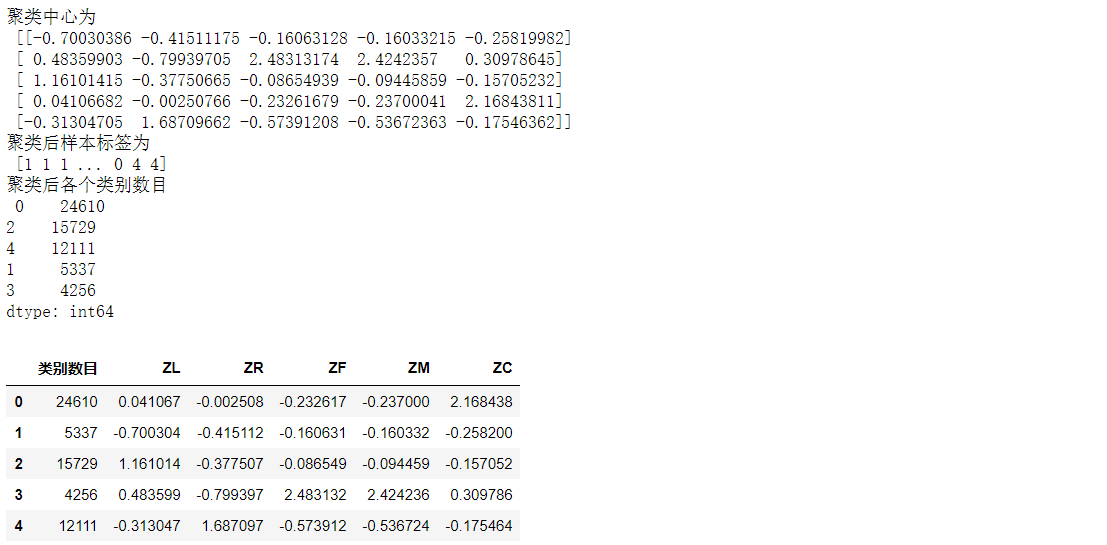### 2.客户价值分析

针对聚类结果进行特征分析，绘制客户分群雷达图

import matplotlib.pyplot as plt
plt.rcParams['font.sans-serif'] = ['SimHei']  # 用来正常显示中文标签
plt.rcParams['axes.unicode_minus'] = False  # 用来正常显示负号

# 客户分群雷达图
labels = ['ZL','ZR','ZF','ZM','ZC']
legen = ['客户群' + str(i + 1) for i in cluster_center.index]  # 客户群命名，作为雷达图的图例
lstype = ['-','--',(0, (3, 5, 1, 5, 1, 5)),':','-.']
kinds = list(cluster_center.index)
# 由于雷达图要保证数据闭合，因此再添加L列，并转换为 np.ndarray
cluster_center = pd.concat([cluster_center, cluster_center[['ZL']]], axis=1)
centers = np.array(cluster_center)

# 分割圆周长，并让其闭合
n = len(labels)
# endpoint=False表示一定没有stop
angle = np.linspace(0, 2 * np.pi, n, endpoint=False)
angle = np.concatenate((angle, [angle]))
# 绘图
fig = plt.figure(figsize = (8,6))
# 以极坐标的形式绘制图形
# 画线
for i in range(len(kinds)):
ax.plot(angle, centers[i], linestyle=lstype[i], linewidth=2,label=kinds[i])
# 添加属性标签
ax.set_thetagrids(angle * 180 / np.pi, labels)
plt.title('客户特征分析雷达图')
plt.legend(legen)
plt.show()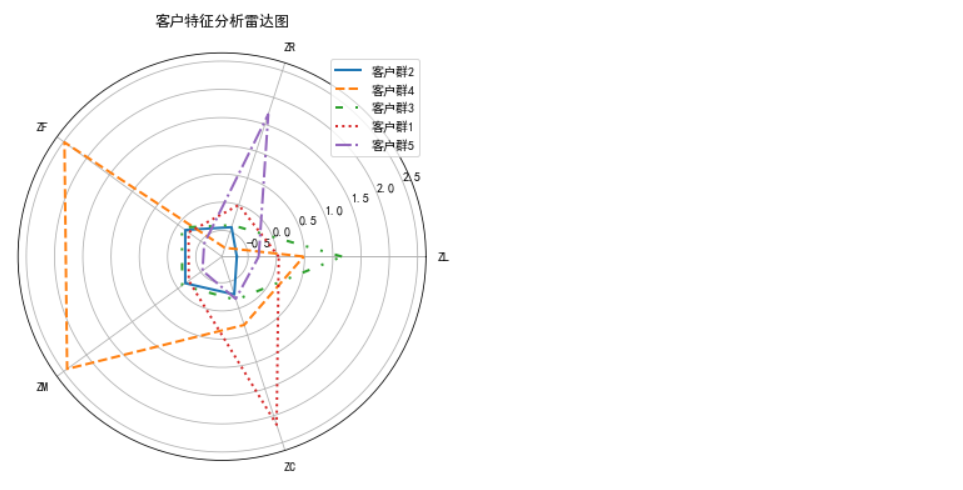总结每个客户群的优势与弱势特征根据以上特征分析，说明不同用户类别的表现特征不同。基于特征描述，我们将客户分为5个等级：重要保持客户，重要发展客户，重要挽留客户，一般客户与低价值客户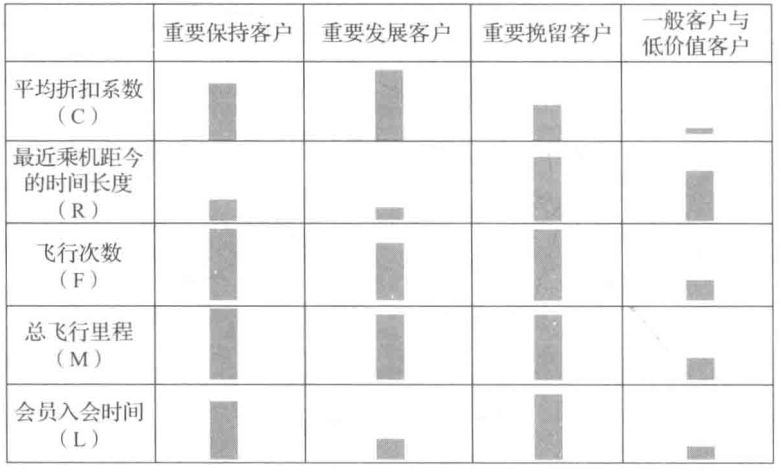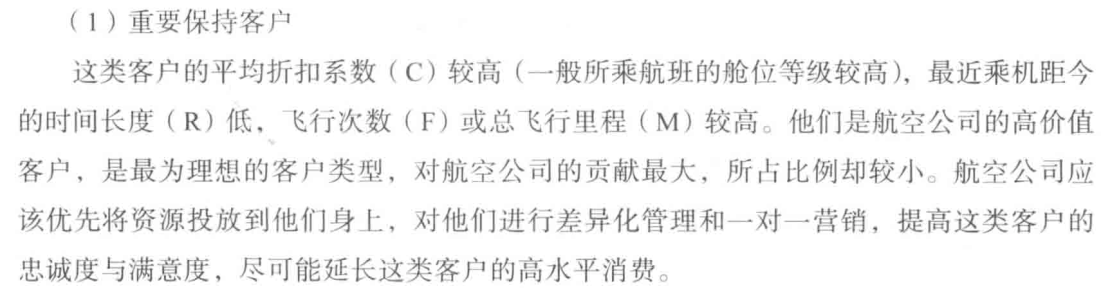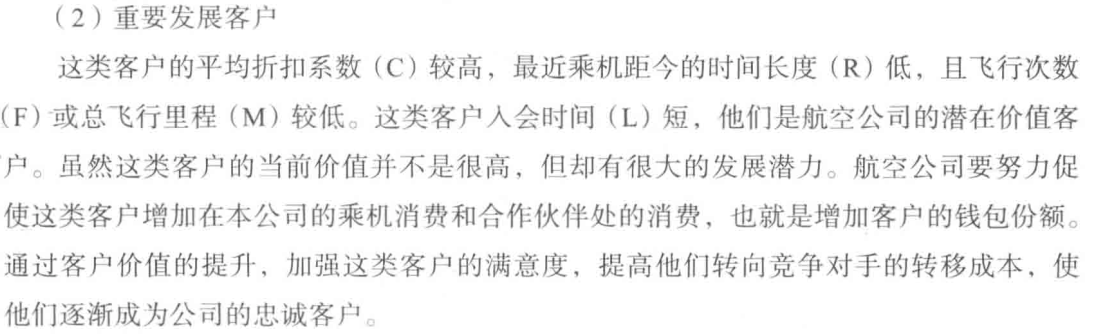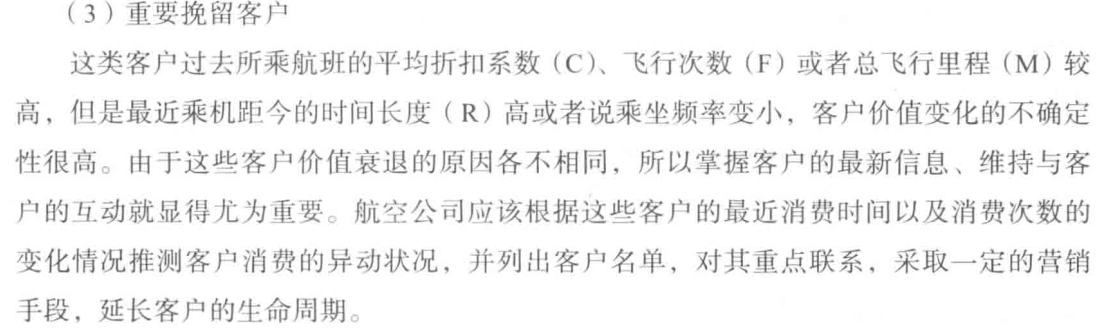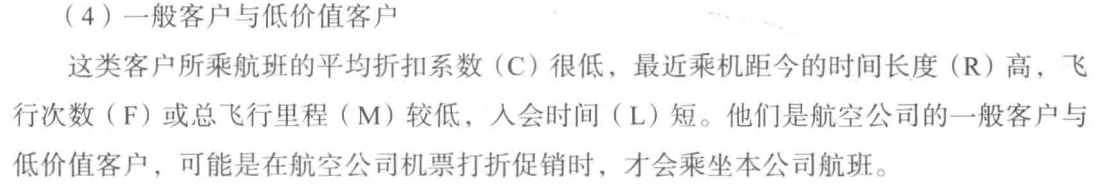05-19562
06-182880
05-121279
12-241214
04-211420
03-021985
04-121274
06-175788
10-281110
07-057104
02-011万+
12-054071
08-198322
11-064227
07-152688
06-092822

### “相关推荐”对你有帮助么？

•非常没帮助
•没帮助
•一般
•有帮助
•非常有帮助

©️2022 CSDN 皮肤主题：Age of Ai 设计师：meimeiellie被折叠的  条评论 为什么被折叠?到【灌水乐园】发言¥2 ¥4 ¥6 ¥10 ¥20余额支付 (余额：-- )扫码支付获取中扫码支付点击重新获取扫码支付1.余额是钱包充值的虚拟货币，按照1:1的比例进行支付金额的抵扣。
2.余额无法直接购买下载，可以购买VIP、C币套餐、付费专栏及课程。余额充值